Online Tution   »   CBSE Sample Paper 2022-23 Class 10   »   CBSE Class 10 Maths Sample Question...

# CBSE Class 10 Maths Sample Paper 2022- 23 with Solution, PDF

## Maths Sample Paper Class 10 2023

CBSE Class 10 Maths Sample Question Paper 2023 is now available on cbseacademic.nic.in .The Central Board of Secondary education has released CBSE Class 10 Sample Question Paper 2023 for all subjects. The CBSE will conduct the Class 10 board exams for the academic year 2022-23 from February 15 onwards. Along with CBSE Class 10 Mathematics Sample Question Paper 2023, CBSE also releases the Marking Scheme of CBSE Class 10 Mathematics Sample Question Paper 2023. So that students can get a clear idea of the board exam format, question kinds, answer options, and other features with the help of CBSE Class 10 Mathematics Sample Question Paper 2023. Here we provide CBSE Class 10 Mathematics Sample Question Paper 2023 in pdf format, Candidates of class 10 can download CBSE Class 10 Mathematics Sample Question Paper 2023 and boost their preparation for the upcoming CBSE Class 10 Board Exam 2023.

Nischay Batch for Class 10 Preparation Online Live Classes by Adda247

Read: CBSE Class 10 Sample Papers 2022-23 for All subjects

## CBSE Maths Class 10 Sample Paper 2022-23 Guidelines

•Time Allowed: 3

•Hours Maximum Marks: 80
General Instructions:
1. This Question Paper has 5 Sections A, B, C, D, and E.
2. Section A has 20 Multiple Choice Questions (MCQs) carrying 1 mark each.
3. Section B has 5 Short Answer-I (SA-I) type questions carrying 2 marks each.
4. Section C has 6 Short Answer-II (SA-II) type questions carrying 3 marks each.
5. Section D has 4 Long Answer (LA) type questions carrying 5 marks each.
6. Section E has 3 Case Based integrated units of assessment (4 marks each) with sub-parts of the values of 1, 1 and 2 marks each respectively.
7. All Questions are compulsory. However, an internal choice in 2 Qs of 2 marks, 2 Qs of 3 marks and 2 Questions of 5 marks has been provided. An internal choice has been provided in the 2 marks questions of Section E.
8. Draw neat figures wherever required. Take π =22/7 wherever required if not stated.

## CBSE Class 10 Maths Sample Paper 2022-23: Section A

Section A

Section A consists of 20 questions of 1 mark each

Q.1.If two positive integers p and q can be expressed as p = ab² and q = a³b; a, b being primenumbers, then LCM (p, q) is
(a) ab          (b) a²b²
(c) a³b²      (d) a³b³

Q.2.What is the greatest possible speed at which a man can walk 52 km and 91 km in an exact
number of hours?
(a) 17 km/hours     (b) 7 km/hours
(c) 13 km/hours     (d) 26 km/hours

Q.3.If one zero of the quadratic polynomial x² + 3x + k is 2, then the value of k is
(a) 10 (b) -10 (c) 5 (d) –5
Q.4.Graphically, the pair of equations given by
6x – 3y + 10 = 0
2x – y + 9 = 0
represents two lines which are
(a) intersecting at exactly one point. (b) parallel.
(c) coincident. (d) intersecting at exactly two points

Q.5. If the quadratic equation x2 + 4x + k = 0 has real and equal roots, then
(a) k < 4 (b) k > 4 (c) k = 4 (d) k ≥ 4

Q.6. The perimeter of a triangle with vertices (0, 4), (0, 0) and (3, 0) is
(a) 5 units (b) 12 units (c) 11 units (d) (7 + √5) units

Q.7.If in triangles ABC and DEF,
AB/DE = BC/FD, then they will be similar, when
(a) ∠B = ∠E   (b) ∠A = ∠D   (c) ∠B = ∠D    (d) ∠A = ∠F

Q.8. In which ratio the y-axis divides the line segment joining the points (5, – 6) and (–1, – 4)?.
(a) 1 : 5      (b) 5 : 1     (c) 1 : 1    (d) 1 : 2

Q.9. In the figure, if PA and PB are tangents to the circle with centre O such that ∠APB = 50°, then ∠OAB is
equal to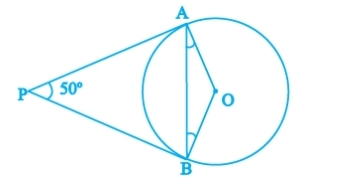(a) 25° (b) 30° (c) 40° (d) 50°

Q.10. If sin A =½, then the value of sec A is :
(a) √3/2      (b) 1√3    (c) √3     (d) 1
Q.11. √3 cos2A + √3 sin2A is equal to
(a) 1   (b) 1√3   (c) √3    (d) 0
Q.12. The value of cos1° cos2° cos3° cos4°…………..…..cos90° is
(a) 1 (b) 0 (c) – 1 (d) 2
Q.13. If the perimeter of a circle is equal to that of a square, then the ratio of their areas is
(a) 22 : 7 (b) 14 : 11 (c) 7 : 22 (d) 11: 14
Q.14. If the radii of two circles are in the ratio of 4 : 3, then their areas are in the ratio of :
(a) 4 : 3 (b) 8 : 3 (c) 16 : 9 (d) 9 : 16
Q.15. The total surface area of a solid hemisphere of radius 7 cm is :
(a) 447π cm²    (b) 239π cm²
(c) 174π cm²      (d) 147π cm²

Q.16.For the following distribution :

 Class 0 – 5 5 – 10 10 – 15 15 – 20 20 – 25 Frequency 10 15 12 20 9

the upper limit of the modal class is
(a) 10 (b) 15 (c) 20 (d) 25
Q.17.If the mean of the following distribution is 2.6, then the value of y is

 Variable (x) 1 2 3 4 5 Frequency 4 5 y 1 2

(a) 3           (b) 8      (c) 13       (d) 24
Q.18.A card is selected at random from a well shuffled deck of 52 cards. The probability of its being a red face card is
(a)3/26  (b) 3/13   (c) 2/13   (d) 1/2

Direction for questions 19 & 20: In question numbers 19 and 20, a statement of Assertion (A) is followed by a statement of Reason (R). Choose the correct option.
Q.19.Assertion: If HCF of 510 and 92 is 2, then the LCM of 510 & 92 is 32460
Reason: as HCF(a,b) x LCM(a,b) = a x b
(a) Both Assertion (A) and Reason (R) are true and Reason (R) is the correct explanation of Assertion (A).
(b) Both Assertion (A) and Reason (R) are true but Reason (R) is not the correct explanation of Assertion (A)
(c) Assertion (A) is true but Reason (R) is false.
(d) Assertion (A) is false but Reason (R) is true.

Q.20. Assertion (A): The ratio in which the line segment joining (2, -3) and (5, 6) internally divided by x axis is 1:2.
Reason (R): as formula for the internal division is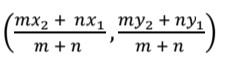(a) Both Assertion (A) and Reason (R) are true and Reason (R) is the correct explanation of Assertion (A).
(b) Both Assertion (A) and Reason (R) are true but Reason (R) is not the correct explanation of Assertion (A).
(c) Assertion (A) is true but Reason (R) is false.
(d) Assertion (A) is false but Reason (R) is true.

## CBSE Class 10 Maths Sample Paper 2022-23: Section B

Section B
Section B consists of 5 questions of 2 marks each.

Q.21. For what values of k will the following pair of linear equations have infinitely many solutions?
kx + 3y – (k – 3) = 0
12x + ky – k = 0

Q.22. In the figure, altitudes AD and CE of Δ ABC intersect each other at the point P. Show that:
(i) ΔABD ~ ΔCBE
(ii) ΔPDC ~ ΔBEC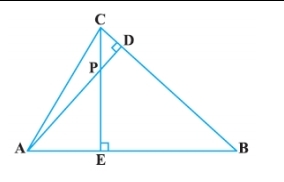[OR]
In the figure, DE || AC and DF || AE. Prove that BF/FE= BE/EC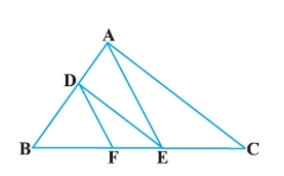Q.23.Two concentric circles are of radii 5 cm and 3 cm. Find the length of the chord of the larger circle which touches the smaller circle.
Q.24. If cot θ =7/8, evaluate (1 + sin θ) (1− sin θ) / (1 + cos θ) (1− cos θ)
Q. 25 Find the perimeter of a quadrant of a circle of radius 14 cm.
[OR]
Find the diameter of a circle whose area is equal to the sum of the areas of the two circles of radii 24 cm and 7 cm.

## CBSE Class 10 Maths Sample Paper 2022-23: Section C

Section C
Section C consists of 6 questions of 3 marks each.

Q.26. Prove that √5 is an irrational number.
Q.27. Find the zeroes of the quadratic polynomial 6x² – 3 – 7x and verify the relationship between the zeroes and the coefficients.
Q.28.A shopkeeper gives books on rent for reading. She takes a fixed charge for the first two days, and an additional charge for each day thereafter. Latika paid Rs 22 for a book kept for six days, while Anand paid Rs 16 for the book kept for four days. Find the fixed charges and the charge for each extra day.
[OR]
Places A and B are 100 km apart on a highway. One car starts from A and another from B at the same time. If the cars travel in the same direction at different speeds, they meet in 5 hours. If they travel towards each other, they meet in 1 hour. What are the speeds of the two cars?

Q.29.In the figure, PQ is a chord of length 8 cm of a circle of radius 5 cm. The tangents at P and Q intersect at point T. Find the length TP.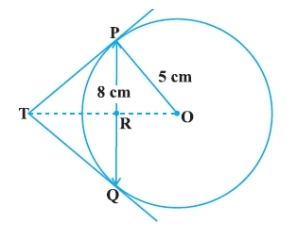Q.30.Prove that, tan θ / (1 − cot θ) + cot θ / (1 − tan θ) = 1 + sec θ cosec θ
[OR]
If sin θ + cos θ = √3, then prove that tan θ + cot θ = 1

Q.31. Two dice are thrown at the same time. What is the probability that the sum of the two numbers appearing on the top of the dice is
(i) 8?
(ii) 13?
(iii) less than or equal to 12?

## CBSE Class 10 Maths Sample Paper 2022-23: Section D

Section D
Section D consists of 4 questions of 5 marks each

Q.32.An express train takes 1 hour less than a passenger train to travel 132 km between Mysore and Bangalore (without taking into consideration the time they stop at intermediate stations). If the average speed of the express train is 11km/h more than that of the passenger train, find the average speed of the two trains.
[OR]
A motor boat whose speed is 18 km/h in still water takes 1 hour more to go 24 km upstream than to return downstream to the same spot. Find the speed of the stream.

Q.33. Prove that If a line is drawn parallel to one side of a triangle to intersect the other two sides in distinct
points, the other two sides are divided in the same ratio. In the figure, find EC if AD/ DB = AE /EC .using the above theorem.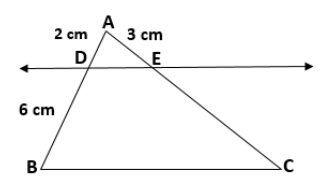Q.34.A pen stand made of wood is in the shape of a cuboid with four conical depressions to hold pens. The
dimensions of the cuboid are 15 cm by 10 cm by 3.5 cm. The radius of each of the depressions is 0.5 cm
and the depth is 1.4 cm. Find the volume of wood in the entire stand.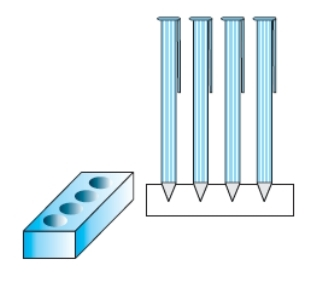[OR]

Ramesh made a bird-bath for his garden in the shape of a cylinder with a hemispherical depression at one
end. The height of the cylinder is 1.45 m and its radius is 30 cm. Find the total surface area of the bird-bath.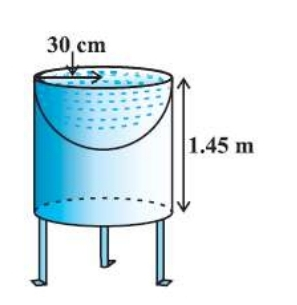Q.35.A life insurance agent found the following data for distribution of ages of 100 policy holders. Calculate the median age, if policies are given only to persons having age 18 years onwards but less than 60 years.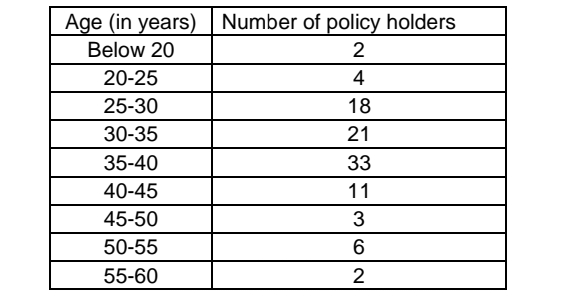## CBSE Class 10 Maths Sample Paper 2022-23: Section E

Section E
Case study-based questions are compulsory.

Q.35.Case Study – 1
In the month of April to June 2022, the exports of passenger cars from India increased by 26% in the corresponding quarter of 2021–22, as per a report. A car manufacturing company planned to produce 1800 cars in 4th year and 2600 cars in 8th year. Assuming that the production increases uniformly by a fixed number every year.Based on the above information answer the following questions

 1 Find the production in the 1st year. 1 II. Find the production in the 12th year. 1 III. Find the total production in first 10 years. [OR] In which year the total production will reach to 15000 cars? 3

Q.37. Case Study – 2
In a GPS, The lines that run east-west are known as lines of latitude, and the lines running north-south are known as lines of longitude. The latitude and the longitude of a place are its coordinates and the distance formula is used to find the distance between two places. The distance between two parallel lines is approximately 150 km. A family from Uttar Pradesh planned a round trip from Lucknow (L) to Puri (P) via Bhuj (B) and Nashik (N) as shown in the given figure below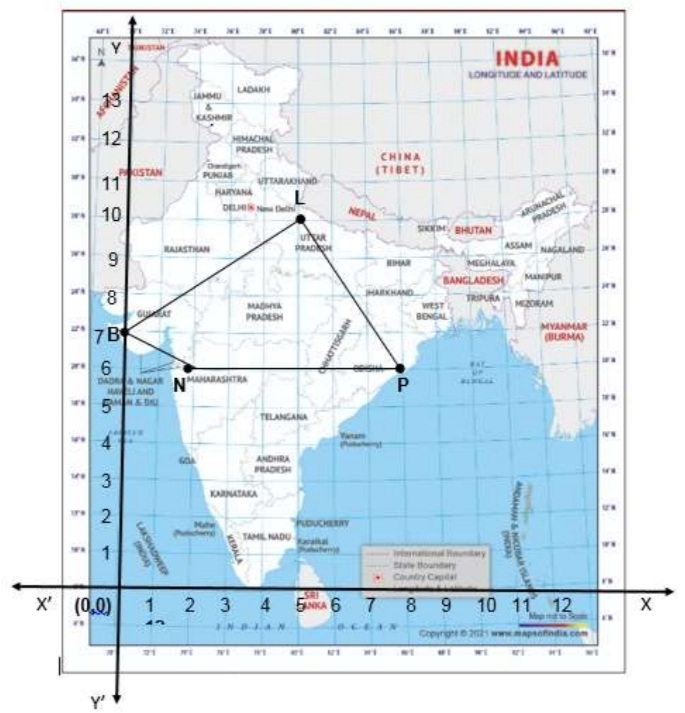Based on the above information answer the following questions using the coordinate geometry.

 I Find the distance between Lucknow (L) to Bhuj(B). 1 II If Kota (K), internally divide the line segment joining Lucknow (L) to Bhuj (B) into 3 : 2 then find the coordinate of Kota (K). 1 III Name the type of triangle formed by the places Lucknow (L), Nashik (N) and Puri (P) [OR] Find a place (point) on the longitude (y-axis) which is equidistant from the points Lucknow (L) and Puri (P). 2

Q.38. Case Study – 3
Lakshaman Jhula is located 5 kilometers northeast of the city of Rishikesh in the Indian state of Uttarakhand.
The bridge connects the villages of Tapovan to Jonk. Tapovan is in Tehri Garhwal district, on the west bank of the river, while Jonk is in Pauri Garhwal district, on the east bank. Lakshman Jhula is a pedestrian bridge also used by motorbikes. It is a landmark of Rishikesh.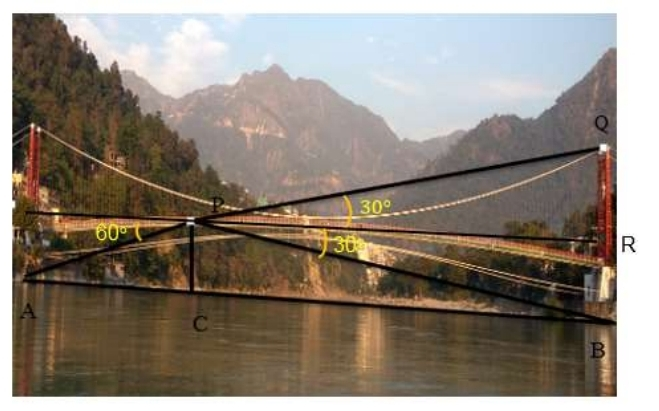Based on the above information answer the following questions.

 Find the distance PA. 1 II. Find the distance PB 1 III. Find the width AB of the river. [OR] Find the height BQ if the angle of the elevation from P to Q be 30° 2

## Class 10 Maths (Standard) Sample Paper 2022-23-SECTION – A

1. Let a and b be two positive integers such that a = p3q4 and b = p2q3 , where p and q are prime numbers. If HCF(a, b) = pmqn and LCM(a, b) = prqs , then (m + n)(r + s) =

(a) 15

(b) 30

(c) 35

(d) 72

2. Let p be a prime number. The quadratic equation having its roots as factors of p is

(a) x2 –px +p=0

(b) x2 –(p+1)x +p=0

(c) x2+(p+1)x +p=0

(d) x2 –px+p+1=0

3. If α and β are the zeros of a polynomial f(x) = px2 – 2x + 3p and α + β = αβ, then p is

(a) -2/3

(b) 2/3

(c) 1/3

(d) -1/3

4. If the system of equations 3x+y =1 and (2k-1)x +(k-1)y =2k+1 is inconsistent, then k =

(a) -1

(b) 0

(c) 1

(d) 2

5. If the vertices of a parallelogram PQRS taken in order are P(3,4), Q(-2,3) and R(-3,-2), then the coordinates of its fourth vertex S are

(a) (-2,-1)

(b) (-2,-3)

(c) (2,-1)

(d) (1,2)

6. ∆ABC~∆PQR. If AM and PN are altitudes of ∆ABC and ∆PQR respectively and AB2 : PQ2 = 4 : 9, then AM: PN =

(a) 3:2

(b) 16:81

(c) 4:9

(d) 2:3

7. If x tan 60° cos 60°= sin60° cot 60° , then x =

(a) cos30°

(b) tan30°

(c) sin30°

(d) cot30°

8. If sinθ + cosθ = √2, then tanθ + cot θ =

(a) 1

(b) 2

(c) 3

(d) 4

9. In the given figure, DE ∥ BC, AE = a units, EC =b units, DE =x units and BC = y units. Which of the following is true?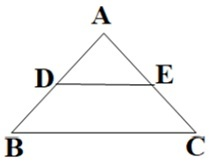(a) x= 𝑎+𝑏/𝑎𝑦

(b) y= 𝑎𝑥/𝑎+𝑏

(c) x= 𝑎𝑦/𝑎+𝑏

(d) 𝑥/𝑦 = 𝑎/b

10. ABCD is a trapezium with AD ∥ BC and AD = 4cm. If the diagonals AC and BD intersect each other at O such that AO/OC = DO/OB =1/2, then BC =

(a) 6cm

(b) 7cm

(c) 8cm

(d) 9cm

11. If two tangents inclined at an angle of 60ᵒ are drawn to a circle of radius 3cm, then the length of each tangent is equal to

(a) 3√3/2 cm

(b) 3cm

(c) 6cm

(d) 3√3cm

12. The area of the circle that can be inscribed in a square of 6cm is

(a) 36π cm2

(b) 18π cm2

(c) 12 π cm2

(d) 9π cm2

13. The sum of the length, breadth and height of a cuboid is 6√3cm and the length of its diagonal is 2√3cm. The total surface area of the cuboid is

(a) 48 cm2

(b) 72 cm2

(c) 96 cm2

(d) 108 cm2

14. If the difference of Mode and Median of a data is 24, then the difference of median and mean is

(a) 8

(b) 12

(c) 24

(d) 36

15. The number of revolutions made by a circular wheel of radius 0.25m in rolling a distance of 11km is

(a) 2800

(b) 4000

(c) 5500

(d) 7000

16. For the following distribution,

 Class 0-5 5-10 10-15 15-20 20-25 Frequency 10 15 12 20 9

the sum of the lower limits of the median and modal class is

(a) 15

(b) 25

(c) 30

(d) 35

17. Two dice are rolled simultaneously. What is the probability that 6 will come up at least once?

(a)1/6

(b) 7/36

(c) 11/36

(d) 13/36

18. If 5 tanβ =4, then 5 𝑠𝑖𝑛𝛽−2 cos𝛽/5 sin𝛽+2 cos𝛽 =

(a) 1/3

(b) 2/5

(c) 3/5

(d) 6

DIRECTION: In the question number 19 and 20, a statement of assertion (A) is followed by a statement of Reason (R). Choose the correct option

19. Statement A (Assertion): If product of two numbers is 5780 and their HCF is 17, then their LCM is 340

Statement R( Reason) : HCF is always a factor of LCM

(a) Both assertion (A) and reason (R) are true and reason (R) is the correct explanation of assertion (A)

(b) Both assertion (A) and reason (R) are true and reason (R) is not the correct explanation of assertion (A)

(c) Assertion (A) is true but reason (R) is false.

(d) Assertion (A) is false but reason (R) is true.

20. Statement A (Assertion): If the co-ordinates of the mid-points of the sides AB and AC of ∆ABC are D(3,5) and E(-3,-3) respectively, then BC = 20 units

Statement R( Reason): The line joining the mid points of two sides of a triangle is parallel to the third side and equal to half of it.

(a) Both assertion (A) and reason (R) are true and reason (R) is the correct explanation of assertion (A)

(b) Both assertion (A) and reason (R) are true and reason (R) is not the correct explanation of assertion (A)

(c) Assertion (A) is true but reason(R) is false.

(d) Assertion (A) is false but reason(R) is true.

## Class 10 Maths (Standard) Sample Paper 2022-23-SECTION – B

21. If 49x+51y= 499, 51 x+49 y= 501, then find the value of x and y

22. In the given figure below, AD/AE = AC/BD and ∠1 = ∠2. Show that ∆ BAE~ ∆CAD.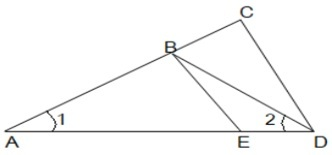23. In the given figure, O is the centre of circle. Find ∠AQB, given that PA and PB are tangents to the circle and ∠APB= 75°.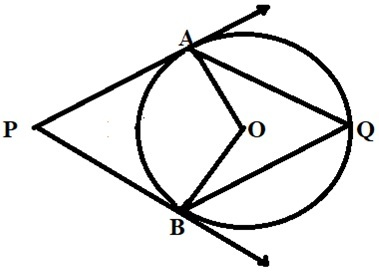24. The length of the minute hand of a clock is 6cm. Find the area swept by it when it moves from 7:05 p.m. to 7:40 p.m.

OR

In the given figure, arcs have been drawn of radius 7cm each with vertices A, B, C and D of quadrilateral ABCD as centres. Find the area of the shaded region.25. If sin(A+B) =1 and cos(A-B)= √3/2, 0°< A+B ≤ 90° and A> B, then find the measures of angles A and B.

OR

Find an acute angle θ when cosθ − sin θ/cosθ + sin θ = 1 − √3/1 + √3

## Class 10 Maths (Standard) Sample Paper 2022-23-SECTION – C

26. Given that √3 is irrational, prove that 5 + 2√3 is irrational.

27. If the zeroes of the polynomial x2 + px +q are double in value to the zeroes of the polynomial 2x2 – 5x – 3, then find the values of p and q.

28. A train covered a certain distance at a uniform speed. If the train would have been 6 km/h faster, it would have taken 4 hours lessthan the scheduled time. And, if the train were slower by 6 km/hr ; it would have taken 6 hours more than the scheduled time. Find the length of the journey.

OR

Anuj had some chocolates, and he divided them into two lots A and B. He sold the first lot at the rate of Rs. 2 for 3 chocolates and the second lot at the rate of Rs. 1 per chocolate, and got a total of Rs. 400. If he had sold the first lot at the rate of Rs. 1 per chocolate, and the second lot at the rate of Rs. 4 for 5 chocolates, his total collection would have been Rs. 460. Find the total number of chocolates he had.

29. Prove the following that-

tan3θ/1+ tan2θ + cot3θ/1 + cot2θ = secθ cosecθ – 2 sinθ cosθ

30. Prove that a parallelogram circumscribing a circle is a rhombus

OR

In the figure XY and X’Y’ are two parallel tangents to a circle with centre O and another tangent AB with point of contact C interesting XY at A and X’Y’ at B, what is the measure of ∠AOB.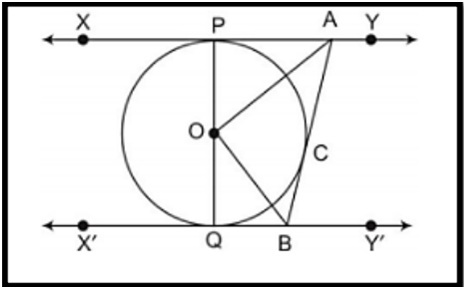31. Two coins are tossed simultaneously. What is the probability of getting

(i) At least one head?

(ii) At most one tail?

(iii) A head and a tail?

## Class 10 Maths (Standard) Sample Paper 2022-23-SECTION – D

32. To fill a swimming pool two pipes are used. If the pipe of larger diameter used for 4 hours and the pipe of smaller diameter for 9 hours, only half of the pool can be filled. Find, how long it would take for each pipe to fill the pool separately, if the pipe of smaller diameter takes 10 hours more than the pipe of larger diameter to fill the pool?

OR

In a flight of 600km, an aircraft was slowed down due to bad weather. Its average speed for the trip was reduced by 200 km/hr from its usual speed and the time of the flight increased by 30 min. Find the scheduled duration of the flight.

33. Prove that if a line is drawn parallel to one side of a triangle intersecting the other two sides in distinct points, then the other two sides are divided in the same ratio.

Using the above theorem prove that a line through the point of intersection of the diagonals and parallel to the base of the trapezium divides the non parallel sides in the same ratio.

34. Due to heavy floods in a state, thousands were rendered homeless. 50 schools collectively decided to provide place and the canvas for 1500 tents and share the whole expenditure equally. The lower part of each tent is cylindrical with base radius 2.8 m and height 3.5 m and the upper part is conical with the same base radius, but of height 2.1 m. If the canvas used to make the tents costs Rs. 120 per m2 , find the amount shared by each school to set up the tents.

OR

There are two identical solid cubical boxes of side 7cm. From the top face of the first cube a hemisphere of diameter equal to the side of the cube is scooped out. This hemisphere is inverted and placed on the top of the second cube’s surface to form a dome. Find

(i) the ratio of the total surface area of the two new solids formed

(ii) volume of each new solid formed.

35. The median of the following data is 525. Find the values of x and y, if the total frequency is 100

 Class interval Frequency 0−100 2 100−200 5 200−300 x 300−400 12 400−500 17 500−600 20 600−700 y 700−800 9 800−900 7 900−1000 4

## Class 10 Maths (Standard) Sample Paper 2022-23-SECTION – E

Case study based questions are compulsory.

36. A tiling or tessellation of a flat surface is the covering of a plane using one or more geometric shapes, called tiles, with no overlaps and no gaps. Historically, tessellations were used in ancient Rome and in Islamic art. You may find tessellation patterns on floors, walls, paintings etc. Shown below is a tiled floor in the archaeological Museum of Seville, made using squares, triangles and hexagons.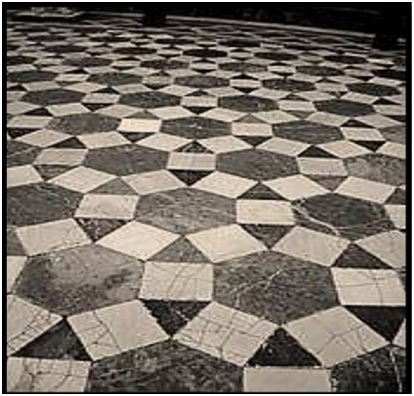A craftsman thought of making a floor pattern after being inspired by the above design. To ensure accuracy in his work, he made the pattern on the Cartesian plane. He used regular octagons, squares and triangles for his floor tessellation pattern.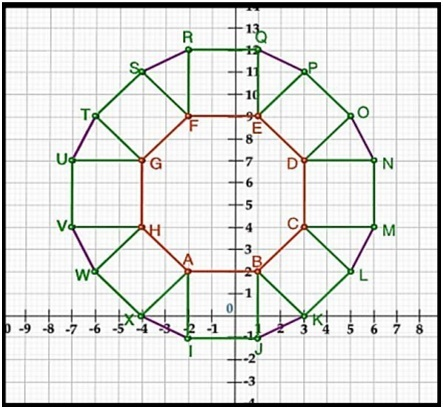Use the above figure to answer the questions that follow:

(i) What is the length of the line segment joining points B and F?

(ii) The centre ‘Z’of the figure will be the point of intersection of the diagonals of quadrilateral WXOP. Then what are the coordinates of Z?

(iii) What are the coordinates of the point on y axis equidistant from A and G?

OR

What is the area of Trapezium AFGH?

37. The school auditorium was to be constructed to accommodate at least 1500 people. The chairs are to be placed in concentric circular arrangement in such a way that each succeeding circular row has 10 seats more than the previous one.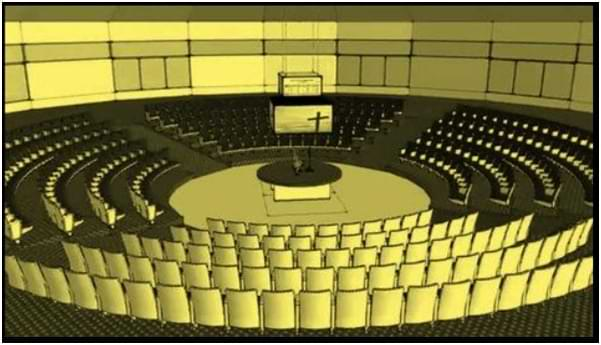(i) If the first circular row has 30 seats, how many seats will be there in the 10th row?

(ii) For 1500 seats in the auditorium, how many rows need to be there?

OR

If 1500 seats are to be arranged in the auditorium, how many seats are still left to be put after 10th row?

(iii) If there were 17 rows in the auditorium, how many seats will be there in the middle row?

38. We all have seen the airplanes flying in the sky but might have not thought of how they actually reach the correct destination. Air Traffic Control (ATC) is a service provided by ground-based air traffic controllers who direct aircraft on the ground and through a given section of controlled airspace, and can provide advisory services to aircraft in non-controlled airspace. Actually, all this air traffic is managed and regulated by using various concepts based on coordinate geometry and trigonometry.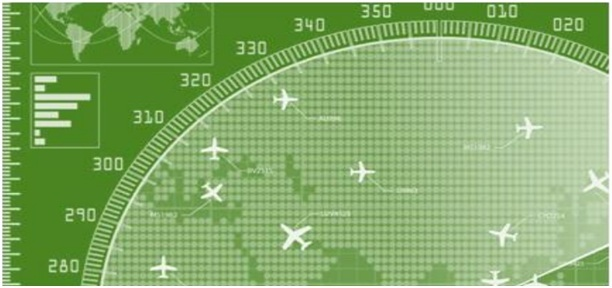At a given instance, ATC finds that the angle of elevation of an airplane from a point on the ground is 60°. After a flight of 30 seconds, it is observed that the angle of elevation changes to 30°. The height of the plane remains constantly as 3000√3 m. Use the above information to answer the questions that follow-

(i) Draw a neat labelled figure to show the above situation diagrammatically.

(ii) What is the distance travelled by the plane in 30 seconds?

OR

Keeping the height constant, during the above flight, it was observed that after 15(√3 -1) seconds, the angle of elevation changed to 45°. How much is the distance travelled in that duration.

(iii) What is the speed of the plane in km/hr.

CBSE Class 10 Mathematics (Standard) Sample Paper 2022-23 in pdf format for you to download and print it according to your convenience.

## Class 10 Maths Sample Paper 2022-23 Pdf Download

Here we are given the  CBSE Class 10 Mathematics Sample Question Paper 2023 in pdf format. Students can download and take a print of CBSE Class 10 Mathematics Sample Question Paper 2023.

## Check CBSE Class 10 Maths  Sample Paper 2022-23 (Basics) PDF Download

CBSE Class 10 Mathematics Sample Question Paper 2023 (Basic) – SQP

## CBSE Class 10 Maths Sample Paper 2022-23: (Basic) Marking Scheme

Check CBSE Class 10 Mathematics Sample Paper 2023 (Basics) Marking Scheme on Adda247

CBSE Class 10 Mathematics Sample Question Paper 2023 (Basics) Marking Scheme

## CBSE Class 10 Maths Sample Paper 2022-23 (Standard): Marking Scheme

Check CBSE Class 10 Mathematics Sample Paper 2023 (Standard) Marking Scheme on Adda247

## CBSE Class 10 Maths Sample Paper 2022-23-FAQs

Q. Where can I get CBSE Class 10 Mathematics Sample Question Paper 2023?

The Central Board of Secondary education has released CBSE Class 10 Mathematics Sample Question Paper 2023 at cbseacademic.nic.in . On this page, we provide CBSE Class 10 Mathematics Sample Question Paper 2023 pdf. You can download it from the page.

Q. When will CBSE conduct CBSE Class 10 Board Examination 2023?

The Central Board of Secondary Education will conduct the CBSE Class 10 Board Examination from 15 February 2023 onwards.

Q.How many sections are there in CBSE Class 10 Mathematics Sample Question Paper 2023 ?

There are a total of five sections present in the CBSE Class 10 Mathematics Sample Question Paper 2023.

1. Section A has 20 Multiple Choice Questions (MCQs) carrying 1 mark each.
2.  Section B has 5 Short Answer-I (SA-I) type questions carrying 2 marks each.
3.  Section C has 6 Short Answer-II (SA-II) type questions carrying 3 marks each.
4.  Section D has 4 Long Answer (LA) type questions carrying 5 marks each.
5.  Section E has 3 Case Based integrated units of assessment (4 marks each) with sub-parts of the values of 1, 1 and 2 marks each respectively.

Sharing is caring!

Thank You, Your details have been submitted we will get back to you.
•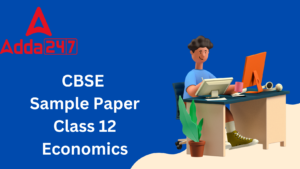CBSE Class 12 Economics Sample Question ...
•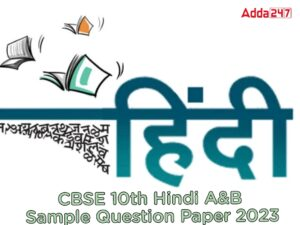CBSE Class 10 Hindi A & B Sample Pap...
•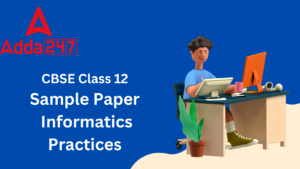CBSE Class 12 Informatics Practices Samp...
•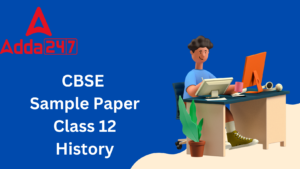CBSE Class 12 History Sample Paper 2022-...
•CBSE Class 12 Maths Sample Paper 2022-23...
•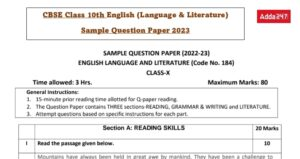Class 10 English Sample Paper 2022- 23 w...